# PSAT Math : Statistics

## Example Questions

### Example Question #43 : How To Find Arithmetic Mean

The average of 12, 11, 10, 16, and x is 12. Solve for x.

9

10

12

11

13

11

Explanation:

The average is the sum of the numbers divided by the total number of terms. Therefore,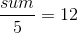.

We can multiply each side by 5 leaving us with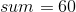,  or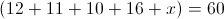.

We can simplify this equation to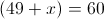.

After subtracting 49 from both sides, we are left with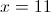.

### Example Question #41 : How To Find Arithmetic Mean

A certain group of 12 students has an average age of 17.  Two new students enter the group.  The average age of the group goes up to 18.  What is the average age of the two new students that came in?

12

20

24

28

16

24

Explanation:

If 12 students have an average age of 17, we can say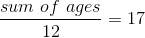.

Therefore the sum of the students' ages is 12 x 17 = 204.

Two students enter the group, so the total number of students goes up to 14.

We are told that the new average age is 18.

Thus, the sum of the ages of the 14 students is 14 x 18 = 252.

The difference of the two sums gives us the sum of the ages of the two new students:

252 - 204 = 48

The average age of the two new students is then 48/2 = 24.

### Example Question #51 : Statistics

On her first four math tests, Kelly earned scores of 75, 85, 92, and 80. What must she score on the fifth test to make her class average 84?

84

83

85

88

90

88

Explanation:

The average of five tests scores must be 84. In other words, (sum of five scores)/5 = 84.

Let's multiply both sides by 5:

sum of five scores = 420

The sum of the first four scores plus the fifth score must be 420. The sum of the first four scores is 75 + 85 + 92 + 80 = 332.

Therefore, 332 + fifth score = 420.

Subtract 332 from both sides:

fifth score = 88.

### Example Question #51 : Data Analysis

A group of four students took a test. The average of their scores was 86. Two students scored 82 on the test. What was the average score of the two other students?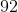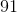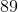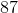Explanation:

We know the average test score was 86, and that the average is the sum of the scores of the students who took the test divided by the number of students.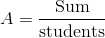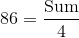To find the sum of the scores on the test, multiply the average score by 4: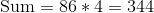The four students scored a total of 344 points on the test. To find the average of the students' scores we don't know, subtract the students' scores we do know from the sum: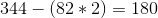The two students whose scores we do not know scored a combined 180 on the test. To find their average score, divide by the number of students whose score we do not know. We are only looking for the average, so their individual scores are not important.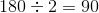The average score of the two students whose score we do not know is 90.

### Example Question #52 : How To Find Arithmetic Mean

On his first three statistics tests, Abe has scored 73, 82, and 75.  What must he score on the fourth test to bring his average to exactly 80?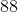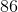Explanation: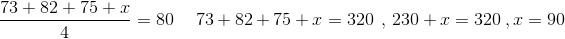### Example Question #1 : How To Find Median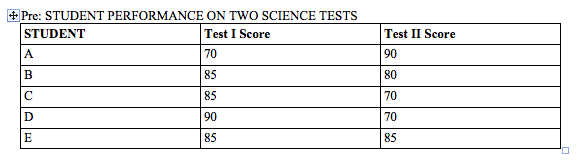The chart above shows the scores on two tests for five students in a science class. If John's score was equal to the mode for Test I and equal to the median for Test II, then which of the five students represents John's scores?

A

D

B

C

E

B

Explanation:

The mode of a set of numbers is the number that appears most frequently in that set. The median of a set of numbers is the number that appears in the middle when they are arranged in numerical order. For Test I, the mode is 85. When arranged in numerical order, the scores for Test II are:

70, 70, 80, 85, 90.

That makes the median for Test II 80. The only student in the chart with an 85 on Test I and an 80 on Test II is student B.

### Example Question #1 : Median

The average (arithmetic mean) of 1, 7, 9, and n is –1. What is the median of this set?

1

7

–2

–4

4

4

Explanation:

If the mean is –1, then 1 + 7 + 9 + n = –4. Solving for n gives us n = –21. In numeric order, we now have –21, 1, 7, 9. As there is an even number of numbers, we average the middle two. (1 + 7) / 2 = 4.

### Example Question #71 : Statistics

The average of 5, 10, 12, 15, and x is 11. What is the median?

13

15

11

12

12

Explanation:

You must first find what x is in order to find the median.

To find x, set up the following equation: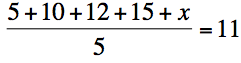To solve the equation, first multiply both sides by 5:

5 + 10 + 12 + 15 + x = 55

Then, add up 5 + 10 + 12 + 15 to get 42:

42 + x = 55

x = 13

Now that you know what x is, you are ready to find the median. To find the median, order the numbers from lowest to highest. The median is the number in the middle.

5, 10, 12, 13, 15

### Example Question #1 : Median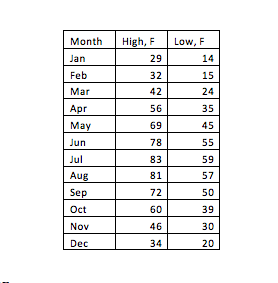The monthly averaged high-and low-temperatures in Michigan are shown in the given table. What are the median monthly averaged high- and low-temperatures respectively in Michigan?

high: 56F, low: 30F

high: 58F, low: 37F

high: 60F, low: 39F

high: 46F, low: 35F

high: 56F, low: 35F

high: 58F, low: 37F

Explanation:

Median is the middle number when the data are ordered from least to greatest. In this case, there are 12 numbers, so, the median is the average of the middle two numbers, which are the 6th and 7th numbers in an ascending order. For the averaged high-temperature, the median is (56+60)/2=58, and for the averaged low-temperature, the median is (35+39)/2=37

### Example Question #1 : How To Find Median

Find the median of the following sequence:

3, 4, 5, 6, 7, 7, 10

6

10

5

7